Larian Studios
Is there a FAQ out there that lists the number of xp points needed for each level?

Not really necessary, I know; just out of interest ... I've been trying to figure out how the % increase per level escalates, but I guess I'm too innumerate.
Yes, Lancet is making a list in his Guide about this matter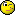Look in his FAQ/Guide and push in CTRL + F together and then type in the searchbox: EXPERIENCE TABLES

Good luckPS:
There must be a logical mathematic behind the XP table, but I search now ...
For DD I have found a formula, with the help of my pc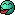(excel )

EDIT :
If I'm correct (with the same kind of formule I found by DD), then the following levels Lancet haven't yet, must be:
Code
```Lvl 28 >   730 800 XP
Lvl 29 >   812 000 XP
Lvl 30 >   899 000 XP
Lvl 31 >   992 000 XP
Lvl 32 > 1 091 200 XP
Lvl 33 > 1 196 800 XP
Lvl 34 > 1 309 000 XP
Lvl 35 > 1 428 000 XP
....
```

If this is correct, then I will explain the formulaThanks, Joram!

I've often looked through Lancet's excellent FAQ, dunno how I missed the xp table - maybe because I was always looking for specific information about items etc ...
Gladly doneI have discovered that the XP table of Ego Draconis have just the same formula as Divine Divinity WITH that difference : by DD you must multiply all by TEN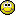I do a try to explain the formula :
I take 2 steps.
First make a 'table' of the subtraction sum of each level.
Step 2 is making the 'table' of all following XP numbers needed to reach the next level, but without the first step, it isn't possible.

STEP 1: table of subtraction sum
Fixed value is always 200, the very first number if you do: 200 XP -(minus) 0 XP = 200
Formula in text: Lvl 1 > take number of first XP value + ( take number of first level and multiply it with fixed value)
Code
``` The Subtraction Table
Lvl 1 > 0 (first XP value) + ( Lvl 1 multiply(*) with 200) = 0 + 200 = 200 (as a first result)
Lvl 2 > 200 (XP) + ( 2 * 200) = 200 + 400 = 600
Lvl 3 > 600 + ( 3 * 200) = 1200
Lvl 4 > 1200 + ( 4 * 200) = 2000
Lvl 5 > 2000 + ( 5 * 200) = 3000
etc ....
```

Now we have taken step 1, we can find the XP needed for the upcoming levelsSTEP 2
Formula in text: take the SUM of all the results of the table in step 1 (the subtraction table) OF the previous levels! This will be more clearly if you see the next table :
Code
```Lvl 1 > just start with 0 XP
Lvl 2 > 0 + 200 (first result by step  1) = 200 XP
Lvl 3 > (zero remains zero in a 'sum') + 200 + 600 = 800 XP
Lvl 4 > 200 + 600 + 1200 = 2000 XP
Lvl 5 > 200 + 600 + 1200 + 2000 = 4000 XP
Lvl 6 > 200 + 600 + 1200 + 2000 + 3000 = 7000 XP
etc ....
```

In a calculating program on a pc you can make fast work of this formulaAnd so you can see I love it to work with numbers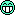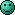Re: xp points per level list? spoilers possible - 06/08/10 03:24 PM

In that case, in D2:ED the experience E required to reach level L is given by the formula below (see the DD topic Wisdom - is it worth the skill points?).

E = 100 * (L^3 - L) / 3
Joram and Raze: wow! and heartfelt thanks. Nothing like having an impenetrable mystery explained ... I often wish I'd been born with a gift for numbers: words are my strong point.

Raze, now I remember that post on DD. After reading it I maxed out Wisdom, and never regretted it.

Haven't had skill points I could spare in DDII yet, though. Ah, well, long way to go.We need to figure out some way to crack the formula for EXP scaling based on character level vs. enemy level, and then some equation to figure out whether Wisdom is a net gain or loss. Seems like the prevailing logic is that "Wisdom means more EXP, which means enemies give less EXP faster," despite the logic problem that if they're giving less EXP faster, it means you leveled faster, which means Wisdom is doing its primary function.

From what I remember of grinding Black Goblins in the Fjords, pre-Battle Tower, EXP scaling maxed out at 3 (or maybe it was 4) levels above you. When you're Lv10, killing a Lv14 enemy would have the same EXP multiplier on the gained EXP as you would get from a Lv20 enemy. Obviously the Lv20 would give more base EXP, but you'd get the same bonus percentage of EXP from each.

I have some old saves, maybe I'll go investigate.
If anyone wants to take a stab at figuring out the EXP scaling from enemies, here's some data. The enemies, if not clear, are Lv18 Black Goblins, Lv19 Black Ring, and Lv21 Imps, all from the early part of Orobas Fjords (around the Grand Knight Shrine and Depleted Ore Mine).

Code
```	Lv18 B. Goblin	Lv19 B. Ring	Lv21 Imp
Lv15	1139		1252		1492
Lv16	1013		1252		1492
Lv17	887		1113		1492
Lv18	761		974		1492
Lv19	761		836		1326
Lv20	534		836		1161
Lv21	281		696		996
Lv22	158		309		996
Lv23	101		174		830
Lv24	70		111		368
Lv25	51		77		207
Lv26	39		56		132
Lv27	31		43		92
Lv28	25		34		67
Lv29	20		27		51
Lv30	17		23		40
Lv31	15		19		33
Lv32	12		16		27```
Re: xp points per level list? spoilers possible - 09/08/10 04:24 AM

Well, if you normalize the experience points by dividing the listed values with that received when your character is at the same level, the level difference modifier is the same for each opponent.

When your character is at a lower level (L) than the enemy (LE), the experience bonus is given by (LE - L)/6 (capped at 0.5, so at 4+ levels below an opponent you get the same experience as 3 levels below). I guess it doesn't help to go after high level opponents too early...
The formula for the experience E given the base experience E' (when your character is at the same level as your opponent) is then:

E = E' (1 + (LE - L)/6 ) when LE-L = 1, 2, 3
E = 1.5 * E' when LE-L > 3

When your character level is more than 1 level above your opponent, the drop off in experience is in the shape of the inverse square of the level difference. A rough formula would be:

E = 3.3 * E' / (L - LE)^2 when L-LE = 2, 3, 4... 14

If I had time to compare the predicted experience points to the observed, maybe a pattern to the discrepancies would emerge, or an explanation for the constant, but for now that's pretty close.